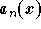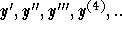#Introduction and First Definitions1.
A differential equation is an equation involving an unknown function and its derivatives.
2.
The order of the differential equation is the order of the highest derivative of the unknown function involved in the equation.
3.
A linear differential equation of order n is a differential equation written in the following form:whereis not the zero function. Note that some may use the notationfor the derivatives.
A linear equation obliges the unknown function y to have some restrictions. Indeed, the only operations which are accepted for the variable y are:

(i)
Differentiating y;
(ii)
Multiplying y and its derivatives by a function of the variable x
(iii)
Adding what you obtained in (ii) and let it be equal to a function of x.

4.
Existence: Does a differential equation have a solution?
5.
Uniqueness: Does a differential equation have more than one solution? If yes, how can we find a solution which satisfies particular conditions?
6.
A problem in which we are looking for the unknown function of a differential equation where the values of the unknown function and its derivatives at some point are known is called an initial value problem (in short IVP).
7.
If no initial conditions are given, we call the description of all solutions to the differential equation the general solution.[Differential Equations] [First Order D.E.]
[Geometry] [Algebra] [Trigonometry ]
[Calculus] [Complex Variables] [Matrix Algebra]S.O.S MATHematics home page

Do you need more help? Please post your question on our S.O.S. Mathematics CyberBoard.Author: Mohamed Amine Khamsi
Last Update 6/22/98